# Isolated Magnetic Island Chain

Suppose that the rigid wall surrounding the plasma is perfectly conducting (i.e.,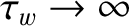, where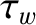is the time constant of the wall—see Section 3.10), and that there is no current flowing through the external magnetic field-coil [i.e.,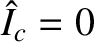, where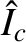is the normalized coil current—see Equation (3.194)]. In this situation, the magnetic island chain that develops at the rational surface is said to be isolated (because it is not subject to any outside influence). For an isolated island chain, Equations (3.187), (3.188), (7.5), as well as the analysis of Section 9.4, lead to the relation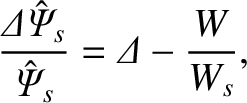(12.1)

where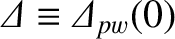is the (real dimensionless) perfect-wall tearing stability index evaluated at zero island width,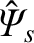the normalized reconnected helical magnetic flux at the rational surface [see Equation (3.184)],the normalized helical sheet current density at the rational surface [see Equation (3.183)],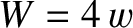the full radial width of the magnetic island chain, and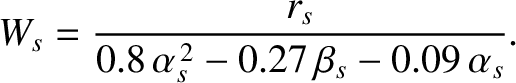(12.2)

Here,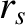is the minor radius of the rational surface, and the dimensionless parameters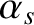and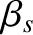are defined in Equations (9.16) and (9.17), respectively. It is clear from the previous equation that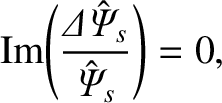(12.3)

which implies that zero electromagnetic torque is exerted at the rational surface. (See Section 3.13.)